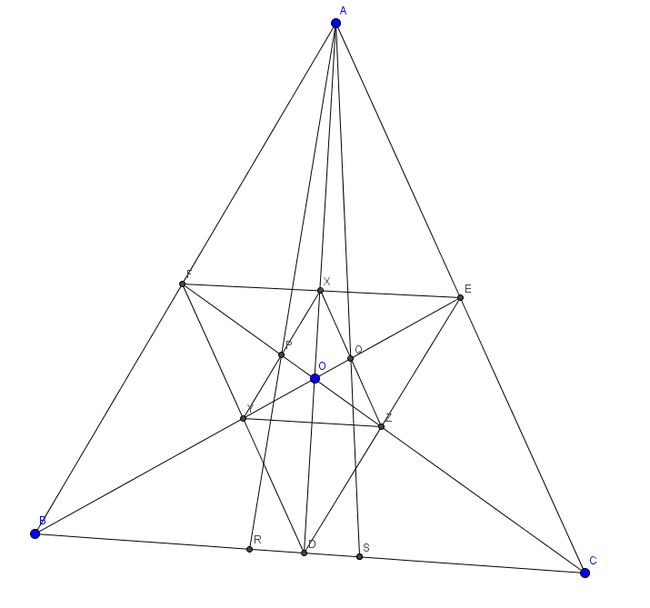# Description of Set

Here are a number of problems based around the same configuration:The solutions I have posted make use of areal/barycentric co-ordinates (two names for the same thing). These are useful in problems involving ratios of lengths, areas and cevians (lines from the verticies of a triangle to the sides that are concurrent at a point).

Full Description

Let $O$ be a point in acute-angle triangle $ABC$.

$D$ is the intersection of $AO$ and $BC$. $E,F$ are defined similarly.

$X$ is the intersection of $EF$ and $AD$. $Y,Z$ are defined similarly.

Let $P$ be the intersection of $XY$ and $CF$ and $Q$ be the intersection of $XZ$ and $BE$.

$R$ is the intersection of $AP$ with $BC$ and $S$ is the intersection of $AQ$ with $BC$.Note by Sam Bealing
5 years, 2 months ago

This discussion board is a place to discuss our Daily Challenges and the math and science related to those challenges. Explanations are more than just a solution — they should explain the steps and thinking strategies that you used to obtain the solution. Comments should further the discussion of math and science.

When posting on Brilliant:

• Use the emojis to react to an explanation, whether you're congratulating a job well done , or just really confused .
• Ask specific questions about the challenge or the steps in somebody's explanation. Well-posed questions can add a lot to the discussion, but posting "I don't understand!" doesn't help anyone.
• Try to contribute something new to the discussion, whether it is an extension, generalization or other idea related to the challenge.
• Stay on topic — we're all here to learn more about math and science, not to hear about your favorite get-rich-quick scheme or current world events.

MarkdownAppears as
*italics* or _italics_ italics
**bold** or __bold__ bold
- bulleted- list
• bulleted
• list
1. numbered2. list
1. numbered
2. list
Note: you must add a full line of space before and after lists for them to show up correctly
paragraph 1paragraph 2

paragraph 1

paragraph 2

[example link](https://brilliant.org)example link
> This is a quote
This is a quote
    # I indented these lines
# 4 spaces, and now they show
# up as a code block.

print "hello world"
# I indented these lines
# 4 spaces, and now they show
# up as a code block.

print "hello world"
MathAppears as
Remember to wrap math in $$ ... $$ or $ ... $ to ensure proper formatting.
2 \times 3 $2 \times 3$
2^{34} $2^{34}$
a_{i-1} $a_{i-1}$
\frac{2}{3} $\frac{2}{3}$
\sqrt{2} $\sqrt{2}$
\sum_{i=1}^3 $\sum_{i=1}^3$
\sin \theta $\sin \theta$
\boxed{123} $\boxed{123}$

## Comments

Sort by:

Top Newest

This looks nice!
Could you explain the configuration in detail, and the results you've obtained?
To me, it looks like the succesive medial triangles of $\triangle ABC$, with common centroid $G$.

- 5 years, 2 months ago

Log in to reply

I've added a full description of the configuration. I've started to write the results I've derived as problems. Here are links to the first two:

- 5 years, 2 months ago

Log in to reply

×

Problem Loading...

Note Loading...

Set Loading...